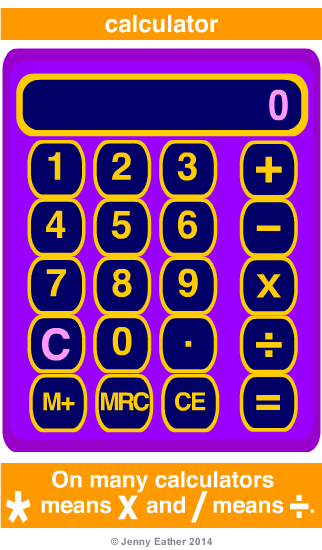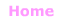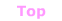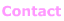Aa Bb Cc Dd Ee Ff Gg Hh Ii Jj Kk Ll Mm Nn Oo Pp Qq Rr Ss Tt Uu Vv Ww Xx Yy Zz

Cc

calculate

• to work something out, a mathematical operation.

mathematical operations

Subtraction (−)
minuend − subtrahend = difference

Multiplication (×)
multiplicand × multiplier = product

Division (÷)
dividend ÷ divisor = quotient

Exponentiation
baseexponent = power

nth root (√)

Logarithm
logbasepower = exponent

calculator

• a calculation device that performs mathematical operations.

EXAMPLE:_______________________________

mathematics calculators online

Including fully explained steps for worked solutions.© Jenny Eather 2014. All rights reserved.